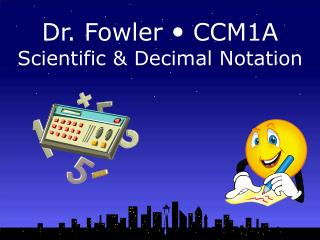# Dr. Fowler  CCM1A Scientific & Decimal Notation - PowerPoint PPT PresentationDownload PresentationDr. Fowler  CCM1A Scientific & Decimal Notation

Dr. Fowler  CCM1A Scientific & Decimal NotationDownload Presentation## Dr. Fowler  CCM1A Scientific & Decimal Notation

- - - - - - - - - - - - - - - - - - - - - - - - - - - E N D - - - - - - - - - - - - - - - - - - - - - - - - - - -
##### Presentation Transcript

1. Dr. Fowler  CCM1A Scientific & Decimal Notation

2. The importance of Scientific Notation: https://www.youtube.com/watch?v=Dme-G4rc6NI

3. How wide is our universe? 210,000,000,000,000,000,000,000 miles (22 zeros) When numbers get this large, it is easier to write them in scientific notation.

4. To write a number in scientific notation: 1. Move the decimal to the right of the first non-zero number. 2. Count how many places the decimal had to be moved. 3. If the decimal had to be moved to the right, the exponent is negative. 4. If the decimal had to be moved to the left, the exponent is positive.

5. Write the width of the universe in scientific notation. 210,000,000,000,000,000,000,000 miles Where is the decimal point now? After the last zero. Where would you put the decimal to make it to the right of the first non-zero number? Between the 2 and the 1

6. 2.10,000,000,000,000,000,000,000. How many decimal places did you move the decimal? 23 Since you moved it left and it is >1, the answer is a positive exponeent 2.1 x 1023

7. 1) Express 0.0000000902 in scientific notation. Where would the decimal go to make it to the right of the first non-zero number? 9.02 The decimal was moved how many places? 8 The decimal was moved to the right, So the exponent is negative. 9.02 x 10-8

8. Try – convert these to Scientific Notation: 4,000 4 X 103 2.48 X 103 2,480 6.123 X 106 6,123,000 306,000,000 3.06 X 108

9. Convert the followingto Decimal Notation: 1.23 X 105 123,000 6.806 X 106 6,806,000

10. In the United States, 15,000,000 households use private wells for their water supply. Write this number in scientific notation. 1.5 X 107

11. The U.S. has a total of 1.2916 X 107 acres of land reserved for state parks. Write this in standard form. 12,916,000 acres

12. Multiplication When multiplying numbers written in scientific notation…..multiply the first factors and add the exponents. Sample Problem: Multiply (3.2 x 10-3) (2.1 x 105) Solution: Multiply 3.2 x 2.1. Add the exponents -3 + 5 Answer: 6.7 x 102

13. Division Divide the numerator by the denominator. Subtract the exponent in the denominator from the exponent in the numerator. Sample Problem: Divide (6.4 x 106) by (1.7 x 102) Solution: Divide 6.4 by 1.7. Subtract the exponents 6 - 2 Answer: 3.8 x 104

14. Addition and Subtraction To add or subtract numbers written in scientific notation, you must….express them with the same power of ten. Sample Problem: Add (5.8 x 103) and (2.16 x 104) Solution: Since the two numbers are not expressed as the same power of ten, one of the numbers will have to be rewritten in the same power of ten as the other. 5.8 x 103 = .58 x 104 so .58 x 104 + 2.16 x 104 =? Answer: 2.74 x 104

15. Excellent Job !!!Well Done